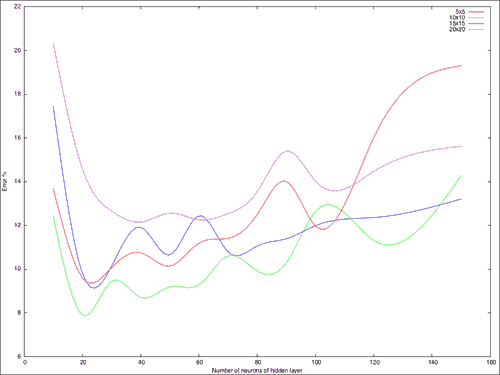• ANPR
• 车牌
• 车牌识别

# ANPR 简介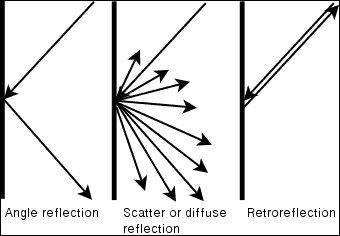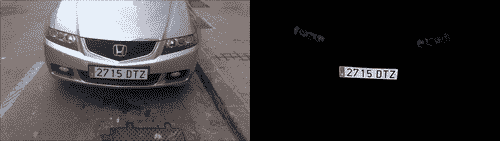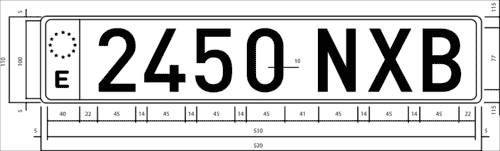# ANPR 算法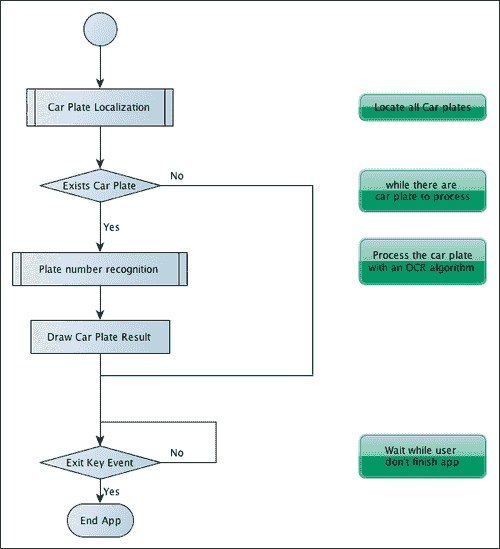1. 分割：此步骤检测并移除图像中每个感兴趣的面片/区域。
2. 特征提取：此步骤从每个补丁中提取一组特征。
3. 分类：此步骤从印版识别步骤中提取每个字符，或在印版检测步骤中将每个图像块分类为“印版”或“无印版”。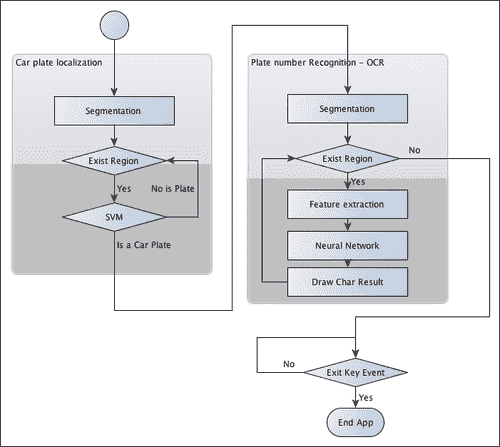• 如何训练模式识别系统
• 如何评估这样的系统

# 车牌检测

• Sobel 过滤器
• 门限操作
• 紧密的形态学操作
• 一个填充区域的遮罩
• 可能检测到的印有红色标记的板（特征图像）
• SVM 分类器后检测到的板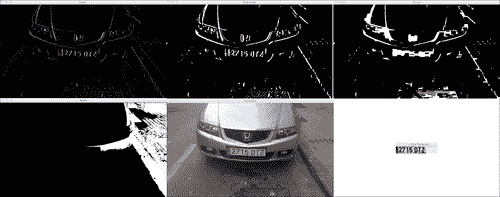## 分割

//convert image to gray
Mat img_gray;
cvtColor(input, img_gray, CV_BGR2GRAY);
blur(img_gray, img_gray, Size(5,5));


void Sobel(InputArray src, OutputArray dst, int ddepth, int xorder, int yorder, int ksize=3, double scale=1, double delta=0, int borderType=BORDER_DEFAULT )


//Find vertical lines. Car plates have high density of vertical lines
Mat img_sobel;
Sobel(img_gray, img_sobel, CV_8U, 1, 0, 3, 1, 0);


//threshold image
Mat img_threshold;
threshold(img_sobel, img_threshold, 0, 255, CV_THRESH_OTSU+CV_THRESH_BINARY);


### 提示

Mat element = getStructuringElement(MORPH_RECT, Size(17, 3));


morphologyEx(img_threshold, img_threshold, CV_MOP_CLOSE, element);


//Find contours of possibles plates
vector< vector< Point> > contours;
findContours(img_threshold,
contours,           // a vector of contours
CV_RETR_EXTERNAL,   // retrieve the external contours
CV_CHAIN_APPROX_NONE); // all pixels of each contour


//Start to iterate to each contour found
vector<vector<Point> >::iterator itc= contours.begin();
vector<RotatedRect> rects;

//Remove patch that has  no inside limits of aspect ratio and area.
while (itc!=contours.end()) {
//Create bounding rect of object
RotatedRect mr= minAreaRect(Mat(*itc));
if( !verifySizes(mr)){
itc= contours.erase(itc);
}else{
++itc;
rects.push_back(mr);
}
}


bool DetectRegions::verifySizes(RotatedRect candidate ){

float error=0.4;
//Spain car plate size: 52x11 aspect 4,7272
const float aspect=4.7272;
//Set a min and max area. All other patches are discarded
int min= 15*aspect*15; // minimum area
int max= 125*aspect*125; // maximum area
//Get only patches that match to a respect ratio.
float rmin= aspect-aspect*error;
float rmax= aspect+aspect*error;

int area= candidate.size.height * candidate.size.width;
float r= (float)candidate.size.width / (float)candidate.size.height;
if(r<1)
r= 1/r;

if(( area < min || area > max ) || ( r < rmin || r > rmax )){
return false;
}else{
return true;
}
}


for(int i=0; i< rects.size(); i++){
//For better rect cropping for each possible box
//Make floodfill algorithm because the plate has white background
//And then we can retrieve more clearly the contour box
circle(result, rects[i].center, 3, Scalar(0,255,0), -1);
//get the min size between width and height
float minSize=(rects[i].size.width < rects[i].size.height)?rects[i].size.width:rects[i].size.height;
minSize=minSize-minSize*0.5;
//initialize rand and get 5 points around center for floodfill algorithm
srand ( time(NULL) );
//Initialize floodfill parameters and variables
mask.create(input.rows + 2, input.cols + 2, CV_8UC1);
int loDiff = 30;
int upDiff = 30;
int connectivity = 4;
int NumSeeds = 10;
Rect ccomp;
int flags = connectivity + (newMaskVal << 8 ) + CV_FLOODFILL_FIXED_RANGE + CV_FLOODFILL_MASK_ONLY;
for(int j=0; j<NumSeeds; j++){
Point seed;
seed.x=rects[i].center.x+rand()%(int)minSize-(minSize/2);
seed.y=rects[i].center.y+rand()%(int)minSize-(minSize/2);
circle(result, seed, 1, Scalar(0,255,255), -1);
int area = floodFill(input, mask, seed, Scalar(255,0,0), &ccomp,   Scalar(loDiff, loDiff, loDiff), Scalar(upDiff, upDiff, upDiff), flags);
}


floodFill函数从种子点开始将具有颜色的连接分量填充到遮罩图像中，并设置要填充的像素与相邻像素或种子像素之间的最大上下亮度/色差：

int floodFill(InputOutputArray image, InputOutputArray mask, Point seed, Scalar newVal, Rect* rect=0, Scalar loDiff=Scalar(), Scalar upDiff=Scalar(), int flags=4 )


newVal参数是我们要在填充时放入图像中的新颜色。 参数loDiffupDiff 是要填充的像素与相邻像素或种子像素之间的最大下部和最大上部亮度/色差。

flag参数是以下各项的组合：

• 低位：这些位包含函数中使用的连接性值 4（默认值）或 8。 连通性确定要考虑像素的哪个邻居。
• 高位：这些可以是 0 或以下值的组合：CV_FLOODFILL_FIXED_RANGECV_FLOODFILL_MASK_ONLY

CV_FLOODFILL_FIXED_RANGE设置当前像素和种子像素之间的差异。 CV_FLOODFILL_MASK_ONLY仅会填充图像遮罩，而不会更改图像本身。

//Check new floodfill mask match for a correct patch.
//Get all points detected for minimal rotated Rect
vector<Point> pointsInterest;
RotatedRect minRect = minAreaRect(pointsInterest);
if(verifySizes(minRect)){
…


//Get rotation matrix
float r= (float)minRect.size.width / (float)minRect.size.height;
float angle=minRect.angle;
if(r<1)
angle=90+angle;
Mat rotmat= getRotationMatrix2D(minRect.center, angle,1);


//Create and rotate image
Mat img_rotated;
warpAffine(input, img_rotated, rotmat, input.size(), CV_INTER_CUBIC);


//Crop image
Size rect_size=minRect.size;
if(r < 1)
swap(rect_size.width, rect_size.height);
Mat img_crop;
getRectSubPix(img_rotated, rect_size, minRect.center, img_crop);


Mat resultResized;
resultResized.create(33,144, CV_8UC3);
resize(img_crop, resultResized, resultResized.size(), 0, 0, INTER_CUBIC);
//Equalize cropped image
Mat grayResult;
cvtColor(resultResized, grayResult, CV_BGR2GRAY);
blur(grayResult, grayResult, Size(3,3));
equalizeHist(grayResult, grayResult);



output.push_back(Plate(grayResult,minRect.boundingRect()));


## 分类

SVM 创建一个或多个用于区分数据每一类的超平面。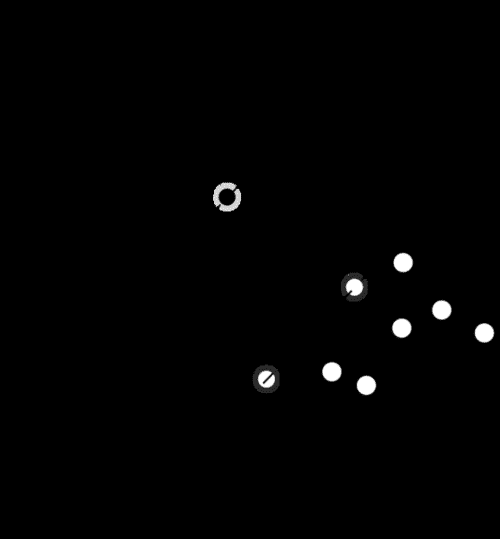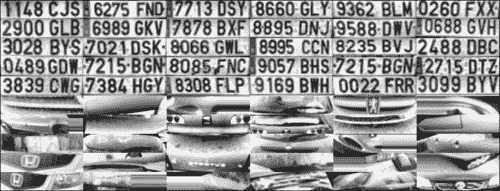### 提示

OpenCV 使用FileStorage类可以轻松地管理 XML 或 JSON 格式的数据文件，该类使我们可以存储和读取 OpenCV 变量和结构或自定义变量。 使用此功能，我们可以读取训练数据矩阵和训练类并将其保存在SVM_TrainingDataSVM_Classes中：

FileStorage fs;
Mat SVM_TrainingData;
Mat SVM_Classes;
fs["TrainingData"] >> SVM_TrainingData;
fs["classes"] >> SVM_Classes;


//Set SVM params
CvSVMParams SVM_params;
SVM_params.kernel_type = CvSVM::LINEAR;


CvSVM svmClassifier(SVM_TrainingData, SVM_Classes, Mat(), Mat(), SVM_params);


vector<Plate> plates;
for(int i=0; i< possible_regions.size(); i++)
{
Mat img=possible_regions[i].plateImg;
Mat p= img.reshape(1, 1);//convert img to 1 row m features
p.convertTo(p, CV_32FC1);
int response = (int)svmClassifier.predict( p );
if(response==1)
plates.push_back(possible_regions[i]);
}


# 车牌识别

## OCR 分割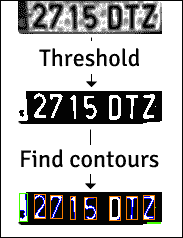Mat img_threshold;
threshold(input, img_threshold, 60, 255, CV_THRESH_BINARY_INV);
if(DEBUG)
imshow("Threshold plate", img_threshold);
Mat img_contours;
img_threshold.copyTo(img_contours);
//Find contours of possibles characters
vector< vector< Point> > contours;
findContours(img_contours,
contours,            // a vector of contours
CV_RETR_EXTERNAL,    // retrieve the external contours
CV_CHAIN_APPROX_NONE); // all pixels of each contour


bool OCR::verifySizes(Mat r)
{
//Char sizes 45x77
float aspect=45.0f/77.0f;
float charAspect= (float)r.cols/(float)r.rows;
float error=0.35;
float minHeight=15;
float maxHeight=28;
//We have a different aspect ratio for number 1, and it can be
//~0.2
float minAspect=0.2;
float maxAspect=aspect+aspect*error;
//area of pixels
float area=countNonZero(r);
//bb area
float bbArea=r.cols*r.rows;
//% of pixel in area
float percPixels=area/bbArea;
if(percPixels < 0.8 && charAspect > minAspect && charAspect <
maxAspect && r.rows >= minHeight && r.rows < maxHeight)
return true;
else
return false;
}


## 特征提取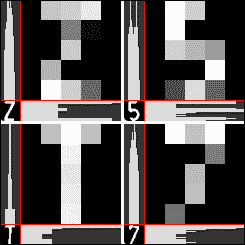Mat OCR::ProjectedHistogram(Mat img, int t)
{
int sz=(t)?img.rows:img.cols;
Mat mhist=Mat::zeros(1,sz,CV_32F);

for(int j=0; j<sz; j++){
Mat data=(t)?img.row(j):img.col(j);
mhist.at<float>(j)=countNonZero(data);
}

//Normalize histogram
double min, max;
minMaxLoc(mhist, &min, &max);

if(max>0)
mhist.convertTo(mhist,-1 , 1.0f/max, 0);

return mhist;
}


Mat OCR::features(Mat in, int sizeData)
{
//Histogram features
Mat vhist=ProjectedHistogram(in,VERTICAL);
Mat hhist=ProjectedHistogram(in,HORIZONTAL);
//Low data feature
Mat lowData;
resize(in, lowData, Size(sizeData, sizeData) );
int numCols=vhist.cols + hhist.cols + lowData.cols *
lowData.cols;
Mat out=Mat::zeros(1,numCols,CV_32F);
//Assign values to feature
int j=0;
for(int i=0; i<vhist.cols; i++)
{
out.at<float>(j)=vhist.at<float>(i);
j++;
}
for(int i=0; i<hhist.cols; i++)
{
out.at<float>(j)=hhist.at<float>(i);
j++;
}
for(int x=0; x<lowData.cols; x++)
{
for(int y=0; y<lowData.rows; y++)
{
out.at<float>(j)=(float)lowData.at<unsigned char>(x,y);
j++;
}
}
return out;
}


## OCR 分类

MLP 由具有输入层，输出层和一个或多个隐藏层的神经元网络组成。 每一层都有一个或多个与上一层和下一层相连的神经元。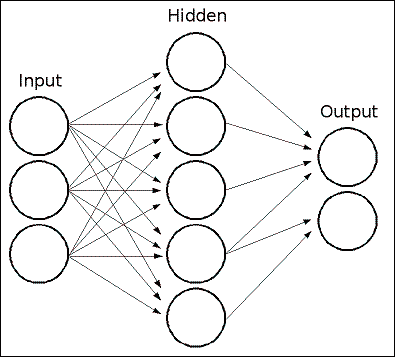MLP 中的所有神经元都是相似的，每个神经元都有多个输入（前一个链接的神经元）和几个具有相同值的输出链接（下一个链接的神经元）。 每个神经元将输出值计算为加权输入加上偏差项的总和，并通过选定的激活函数进行转换：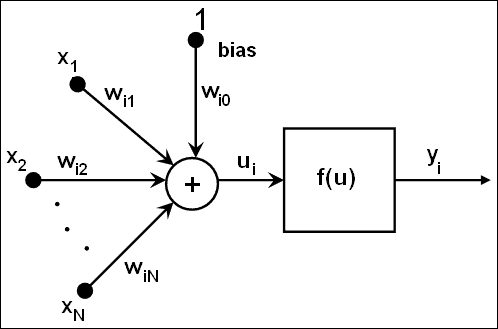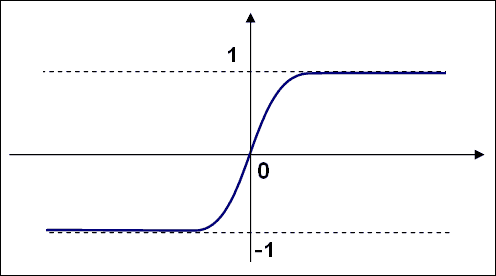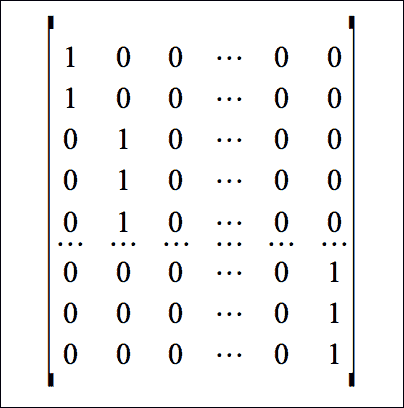OpenCV 为 ANN 定义了一个CvANN_MLP类。 使用create函数，我们可以通过定义层和神经元的数量，激活函数以及alphabeta参数来启动类：

void OCR::train(Mat TrainData, Mat classes, int nlayers)
{
Mat layerSizes(1,3,CV_32SC1);
layerSizes.at<int>(0)= TrainData.cols;
layerSizes.at<int>(1)= nlayers;
layerSizes.at<int>(2)= numCharacters;
ann.create(layerSizes, CvANN_MLP::SIGMOID_SYM, 1, 1); //ann is
global class variable

//Prepare trainClasses
//Create a mat with n trained data by m classes
Mat trainClasses;
trainClasses.create( TrainData.rows, numCharacters, CV_32FC1 );
for( int i = 0; i <  trainClasses.rows; i++ )
{
for( int k = 0; k < trainClasses.cols; k++ )
{
//If class of data i is same than a k class
if( k == classes.at<int>(i) )
trainClasses.at<float>(i,k) = 1;
else
trainClasses.at<float>(i,k) = 0;
}
}
Mat weights( 1, TrainData.rows, CV_32FC1, Scalar::all(1) );

//Learn classifier
ann.train( TrainData, trainClasses, weights );
trained=true;
}


int OCR::classify(Mat f)
{
int result=-1;
Mat output(1, numCharacters, CV_32FC1);
ann.predict(f, output);
Point maxLoc;
double maxVal;
minMaxLoc(output, 0, &maxVal, 0, &maxLoc);
//We need to know where in output is the max val, the x (cols) is
//the class.

return maxLoc.x;
}


CvANN_MLP类使用predict函数对类中的特征向量进行分类。 与 SVM classify函数不同，ANN 的predict函数返回一行，其大小等于类的数量，并且有可能属于每个类的输入特征。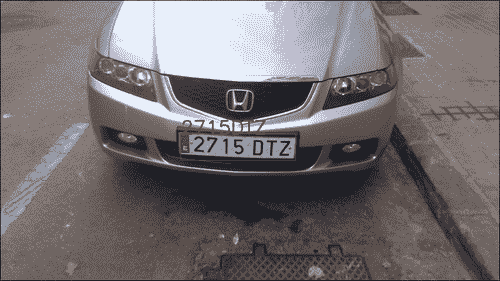string licensePlate=plate.str();
rectangle(input_image, plate.position, Scalar(0,0,200));
putText(input_image, licensePlate, Point(plate.position.x, plate.position.y), CV_FONT_HERSHEY_SIMPLEX, 1, Scalar(0,0,200),2);


## 评估

Mat classes;
Mat trainingData;
FileStorage fs;
fs[data] >> trainingData;
fs["classes"] >> classes;


float test(Mat samples, Mat classes)
{
float errors=0;
for(int i=0; i<samples.rows; i++)
{
int result= ocr.classify(samples.row(i));
if(result!= classes.at<int>(i))
errors++;
}
return errors/samples.rows;
}


#!/bin/bash
echo "#ITS \t 5 \t 10 \t 15 \t 20" > data.txt
folder=$(pwd) for numNeurons in 10 20 30 40 50 60 70 80 90 100 120 150 200 500 do s5=0; s10=0; s15=0; s20=0; for j in {1..100} do echo$numNeurons $j a=$($folder/build/evalOCR$numNeurons TrainingDataF5)
s5=$(echo "scale=4;$s5+$a" | bc -q 2>/dev/null) a=$($folder/build/evalOCR$numNeurons TrainingDataF10)
s10=$(echo "scale=4;$s10+$a" | bc -q 2>/dev/null) a=$($folder/build/evalOCR$numNeurons TrainingDataF15)
s15=$(echo "scale=4;$s15+$a" | bc -q 2>/dev/null) a=$($folder/build/evalOCR$numNeurons TrainingDataF20)
s20=$(echo "scale=4;$s20+$a" | bc -q 2>/dev/null) done echo "$i \t $s5 \t$s10 \t $s15 \t$s20"
echo "$i \t$s5 \t $s10 \t$s15 \t \$s20" >> data.txt
done